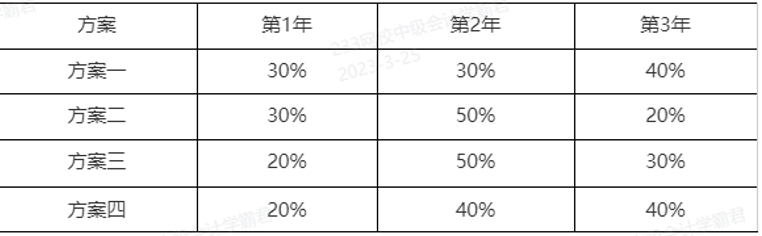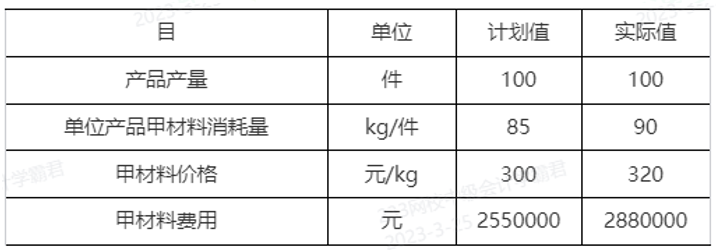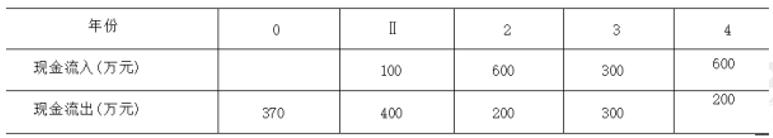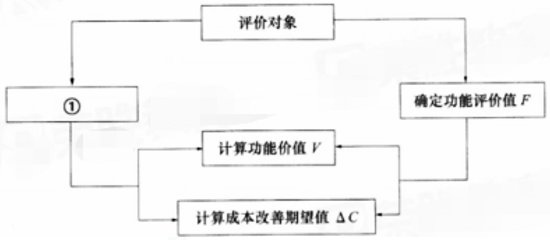100:002022年补考《建设工程经济》真题

1
(单项选择题)

• A.

综合单价法

• B.

参数法

• C.

工料单价法

• D.

分包法

• A
• B
• C
• D

2
(单项选择题)

• A.

270

• B.

300

• C.

324

• D.

320

• A
• B
• C
• D

3
(单项选择题)

• A.

财产保险费

• B.

规费

• C.

企业管理费

• D.

社会保险费

• A
• B
• C
• D

4
(单项选择题)

• A.

保险费

• B.

劳动保护费

• C.

进场临时道路费

• D.

经营业务费

• A
• B
• C
• D

5
(单项选择题)

• A.

分组计算审查法

• B.

标准预算审查法

• C.

对比审查法

• D.

筛选审查法

• A
• B
• C
• D

6
(单项选择题)

• A.

76.25

• B.

126.25

• C.

200.00

• D.

101.25

• A
• B
• C
• D

7
(单项选择题)

• A.

971.93

• B.

981.04

• C.

1000.00

• D.

1092.10

• A
• B
• C
• D

8
(单项选择题)

• A.

10.25%

• B.

10.38%

• C.

10.43%

• D.

10.50%

• A
• B
• C
• D

9
(单项选择题)

• A.

科学技术进步越快，设备的技术寿命越短

• B.

设备的技术寿命主要由设备的有形磨损决定

• C.

设备更新应主要考虑其自然寿命

• D.

设备的自然寿命主要由设备的无形磨损决定

• A
• B
• C
• D

10
(单项选择题)• A.

方案一

• B.

方案二

• C.

方案三

• D.

方案四

• A
• B
• C
• D

11
(单项选择题)

• A.

总投资收益率

• B.

投资回收期

• C.

财务内部收益率

• D.

利息备付率

• A
• B
• C
• D

12
(单项选择题)

• A.

财务基准收益率的确定与技术方案的特点无关

• B.

财务基准收益率的确定应考虑投资的机会成本

• C.

财务基准收益率的确定不应考虑通货膨胀的影响

• D.

投资风险较高的技术方案可适当降低财务基准收益率

• A
• B
• C
• D

13
(单项选择题)• A.

18

• B.

15

• C.

16

• D.

17

• A
• B
• C
• D

14
(单项选择题)

• A.

对承包人已完成的合同范围内且质量达到合同标准的工程进行计量

• B.

对承包人已完成的全部工程进行计量

• C.

对因清单中出现工程量计算偏差引起的工程量增加不予计量

• D.

对因承包人原因造成的返工工程量应予以计量

• A
• B
• C
• D

15
(单项选择题)

• A.

费用定额

• B.

概算定额

• C.

预算定额

• D.

施工定额

• A
• B
• C
• D

16
(单项选择题)

• A.

建设期内材料价格上涨增加的费用

• B.

设计变更及施工过程中可能增加工程量的费用

• C.

因施工质量不合格返工增加的费用

• D.

因业主方拖欠工程款增加的承包方借款利息

• A
• B
• C
• D

17
(单项选择题)

• A.

总概算价值＝工程费用＋其他费用＋预备费＋铺底流动资金－回收金额

• B.

总概算价值＝工程费用＋其他费用＋预备费＋流动资金－回收金额

• C.

总概算价值＝工程费用＋其他费用＋预备费＋建设期利息＋铺底流动资金－回收金额

• D.

总概算价值＝工程费用＋其他费用＋预备费＋建设期利息＋流动资金

• A
• B
• C
• D

18
(单项选择题)

• A.

技术方案的资本金后于负债受偿

• B.

投资者可以以任何方式抽回资本金

• C.

技术方案资本金只能以现金方式出资

• D.

技术方案资本金是指企业实体的注册资

• A
• B
• C
• D

19
(单项选择题)

• A.

承包人提供质量保证金的方式原则上采用扣留相应比例工程款的方式

• B.

在工程竣工前，承包人已提供履约担保的，发包人不得同时预留质量保证金

• C.

在支付工程进度款时逐次扣留质量保证金的，质量保证金的计算基数应包括每次价格调整的金额

• D.

发包人累计扣留的质量保证金不得超过工程价款结算总额的5%

• A
• B
• C
• D

20
(单项选择题)

• A.

结构工程中的现浇混凝土强度

• B.

方案占地面积

• C.

施工专业化协作能保证供应

• D.

施工方案可能形成的污染情况

• A
• B
• C
• D

21
(单项选择题)

• A.

未通知承包人到场，自行抽样复测并修正结果

• B.

根据发包人和承包人协商结果修正工程量

• C.

要求承包人自行复核并据此修正结果

• D.

要求承包人共同复核或抽样复测

• A
• B
• C
• D

22
(单项选择题)

• A.

决策进度和设计阶段

• B.

招投标和施工阶段

• C.

设计和招投标阶段

• D.

施工和运营阶段

• A
• B
• C
• D

23
(单项选择题)

• A.

6988.50

• B.

6688.50

• C.

6880.0

• D.

6888.50

• A
• B
• C
• D

24
(单项选择题)

• A.

投标文件

• B.

造价咨询机构确认书

• C.

招标文件

• D.

审计报告

• A
• B
• C
• D

25
(单项选择题)

• A.

重要性评分比例

• B.

寿命期比例

• C.

制造成本比例

• D.

公允价值比例

• A
• B
• C
• D

26
(单项选择题)

• A.

当工程量减少15%以上时，减少后剩余部分的工程量综合单价应予调整

• B.

当工程量减少不超过20%时，减少后剩余部分的工程量综合单价不予调整

• C.

因工程量变化引起的措施项目费一律不予调整

• D.

对工程量增加15%以上的部分其综合单价应予调高

• A
• B
• C
• D

27
(单项选择题)

• A.

70

• B.

900

• C.

600

• D.

1000

• A
• B
• C
• D

28
(单项选择题)

• A.

预算定额基价一般不随市场价格变化而变化

• B.

预算定额基价以全国平均价格水平为基础进行编制

• C.

编制预算定额基价时，项目的划分、名称、编号可自行设定

• D.

预算定额基价只包括人工费、材料费和施工机具使用费

• A
• B
• C
• D

29
(单项选择题)

• A.

专项评价费

• B.

勘察设计费

• C.

研究试验费

• D.

设备检验费

• A
• B
• C
• D

30
(单项选择题)

• A.

100000

• B.

148250

• C.

147500

• D.

150000

• A
• B
• C
• D

31
(单项选择题)

• A.

资产负债率

• B.

销售净利率

• C.

净资产收益率

• D.

总资产周转率

• A
• B
• C
• D

32
(单项选择题)

• A.

暂估价中的材料可以按照暂估单价计入综合单价，也可以按照自行采购单价（比暂估价低）计入综合单价

• B.

计日工应按照招标工程量清单列出的项目和估算的数量计算，自主确定综合单价，但不计入总报价

• C.

专业工程暂估价应按照招标工程量清单中列出的金额填写，不得变动

• D.

总承包服务费根据合同计价模式按照固定的费率计取，与招标人的具体要求关系不大

• A
• B
• C
• D

33
(单项选择题)

• A.

1.67%

• B.

15%

• C.

20.71%

• D.

21.67%

• A
• B
• C
• D

34
(单项选择题)

• A.

3.7

• B.

6.47

• C.

4.6

• D.

6.25

• A
• B
• C
• D

35
(单项选择题)• A.

财务净现值为-37.26万元，方案不可行

• B.

财务净现值为-10.83万元，方案不可行

• C.

财务净现值为13.64万元，方案可行

• D.

财务净现值为18.57万元，方案可行

• A
• B
• C
• D

36
(单项选择题)

• A.

功能整理——功能定义——功能评价——功能成本分析

• B.

功能定义——功能评价——功能整理——功能成本分析

• C.

功能整理——功能定义——功能成本分析——功能评价

• D.

功能定义——功能整理——功能成本分析——功能评价

• A
• B
• C
• D

37
(单项选择题)

• A.

承包人承担

• B.

发包人承担

• C.

监理人承担

• D.

发包人和承包人各承担一半

• A
• B
• C
• D

38
(单项选择题)

• A.

5.36%

• B.

6.24%

• C.

7.00%

• D.

7.15%

• A
• B
• C
• D

39
(单项选择题)

• A.

314.81

• B.

370.37

• C.

385.19

• D.

355.56

• A
• B
• C
• D

40
(单项选择题)

• A.

施工合同收入

• B.

让渡资产使用权收入

• C.

提供劳务收入

• D.

销售商品收入

• A
• B
• C
• D

41
(单项选择题)

• A.

最终结算申请之前分生的所有索赔

• B.

工程接收证书颁发之后发生的索赔

• C.

竣工结算之前发生的索赔

• D.

竣工结算审核到接受竣工付款证书期间发生的索赔

• A
• B
• C
• D

42
(单项选择题)

• A.

港口费

• B.

样品费

• C.

采购保管损耗费

• D.

银行手续费

• A
• B
• C
• D

43
(单项选择题)

• A.

36.00

• B.

22.80

• C.

24.77

• D.

27.74

• A
• B
• C
• D

44
(单项选择题)

• A.

交易性

• B.

投机性

• C.

预防性

• D.

风险管理

• A
• B
• C
• D

45
(单项选择题)

• A.

建设单位支付工资不超过1个月

• B.

可以以实物或者有价证券，支付给本人

• C.

施工单位同意他人个人或者单位招揽工程，拖欠工资的，由施工单位支付工人工资

• D.

建设工程违反法律法规而拖欠农民工工资的由建设单位支付工人工资

• A
• B
• C
• D

46
(单项选择题)

• A.

20.25

• B.

32.91

• C.

57.29

• D.

35.26

• A
• B
• C
• D

47
(单项选择题)

• A.

招标工程量清单中的工程量应由投标人结合自己的施工方案进行调整，以合同约定为准

• B.

招标工程量清单中工程量可作为承包人在履行合同义务中实际完成的准确工程量

• C.

发承包双方进行工程竣工结算时的工程数量，应以承包方提供的工程量为准

• D.

招标工程量清单中的工程量是工程量清单编制人按施工图图示尺寸和工量计算规则得到的工程净量

• A
• B
• C
• D

48
(单项选择题)• A.

定义功能

• B.

确定现实成本

• C.

确定目标成本

• D.

进行功能分析

• A
• B
• C
• D

49
(单项选择题)

• A.

将拟实施的方案提交发包人确认，并详细说明变化情况

• B.

计算报价浮动率，据此计算调整后的措施项目费

• C.

提交安全文明施工费的计算依据和浮动比率

• D.

将措施项目费估算的方法提交监理人确认

• A
• B
• C
• D

50
(单项选择题)

• A.

确认收入的同一时期

• B.

收入实际到账的时期

• C.

成本和费用实际发生的时期

• D.

成本和费用发生当年的年末

• A
• B
• C
• D

51
(单项选择题)

• A.

32.00%

• B.

53.33%

• C.

34.78%

• D.

92.00%

• A
• B
• C
• D

52
(单项选择题)

• A.

股利取决于盈利水平，无固定股利负担

• B.

不会改变股东对公司的控制权

• C.

作为公司永久性资金无需归还

• D.

股利取决于分配政策并定期支付

• A
• B
• C
• D

53
(单项选择题)

• A.

①②③④

• B.

③②①④

• C.

④③②①

• D.

④②①③

• A
• B
• C
• D

54
(单项选择题)

• A.

多余和偶然的工作时间

• B.

有效工作时间

• C.

不可避免的中断时间

• D.

休息时间

• A
• B
• C
• D

55
(单项选择题)

• A.

确认为支出当期产生的费用

• B.

列入支出当期的营业成本

• C.

列入支出当期的待核销支出

• D.

从当期的利润总额中扣除

• A
• B
• C
• D

56
(单项选择题)

• A.

因基准日期后合同适用的法律或政府有关规定发生变化，增加的安全文明施工费由承包人承担

• B.

承包人经发包人同意采取合同预定以外的安全措施所产生的费用，由承包人承担

• C.

发包人应在开工后28天内预付安全文明施工费总额的30%，其余部分与进度款同期支付

• D.

承包人对安全文明施工费应专款专用，并在财务账目中单独列项备查

• A
• B
• C
• D

57
(单项选择题)

• A.

监理人未在收到承包人提交的工程量报表后的7天内完成审核的，则该工程量视为承包人实际完成的工程量

• B.

发包人可以在任何方便的时候计量，计量结果有效

• C.

承包人收到计量的通知后不派人参加，则发包人的计量结果无效

• D.

承包人为保证施工质量超出施工图纸范围的工程量，监理人应全部予以计量

• A
• B
• C
• D

58
(单项选择题)

• A.

150.00

• B.

300.00

• C.

474.34

• D.

670.82

• A
• B
• C
• D

59
(单项选择题)

• A.

工程造价管理机构通过工程造价信息发布的材料单价

• B.

当时国际市场的材料单价

• C.

近三个月当地已完工程材料结算单价的平均值

• D.

招标人的材料供应商提供的材料单价

• A
• B
• C
• D

60
(单项选择题)

• A.

计算临界点

• B.

估计不确定因素的变化幅度

• C.

确定分析指标

• D.

确定风险概率

• A
• B
• C
• D